Open in App
Not now

# Ukkonen’s Suffix Tree Construction – Part 4

• Difficulty Level : Expert
• Last Updated : 28 Nov, 2021

Ukkonen’s Suffix Tree Construction – Part 1
Ukkonen’s Suffix Tree Construction – Part 2
Ukkonen’s Suffix Tree Construction – Part 3

Please go through Part 1, Part 2 and Part 3, before looking at current article, where we have seen few basics on suffix tree, high level ukkonen’s algorithm, suffix link and three implementation tricks and some details on activePoint along with an example string “abcabxabcd” where we went through four phases of building suffix tree.

Let’s revisit those four phases we have seen already in Part 3, in terms of trick 2, trick 3 and activePoint.

• activePoint is initialized to (root, NULL, 0), i.e. activeNode is root, activeEdge is NULL (for easy understanding, we are giving character value to activeEdge, but in code implementation, it will be index of the character) and activeLength is ZERO.
• The global variable END and remainingSuffixCount are initialized to ZERO

*********************Phase 1*********************************
In Phase 1, we read 1st character (a) from string S

• Set END to 1
• Increment remainingSuffixCount by 1 (remainingSuffixCount will be 1 here, i.e. there is 1 extension left to be performed)
• Run a loop remainingSuffixCount times (i.e. one time) as below:
• If activeLength is ZERO, set activeEdge to the current character (here activeEdge will be ‘a’). This is APCFALZ.
• Check if there is an edge going out from activeNode (which is root in this phase 1) for the activeEdge. If not, create a leaf edge. If present, walk down. In our example, leaf edge gets created (Rule 2).
• Once extension is performed, decrement the remainingSuffixCount by 1
• At this point, activePoint is (root, a, 0)

At the end of phase 1, remainingSuffixCount is ZERO (All suffixes are added explicitly).
Figure 20 in Part 3 is the resulting tree after phase 1.

*********************Phase 2*********************************
In Phase 2, we read 2nd character (b) from string S

• Set END to 2 (This will do extension 1)
• Increment remainingSuffixCount by 1 (remainingSuffixCount will be 1 here, i.e. there is 1 extension left to be performed)
• Run a loop remainingSuffixCount times (i.e. one time) as below:
• If activeLength is ZERO, set activeEdge to the current character (here activeEdge will be ‘b’). This is APCFALZ.
• Check if there is an edge going out from activeNode (which is root in this phase 2) for the activeEdge. If not, create a leaf edge. If present, walk down. In our example, leaf edge gets created.
• Once extension is performed, decrement the remainingSuffixCount by 1
• At this point, activePoint is (root, b, 0)

At the end of phase 2, remainingSuffixCount is ZERO (All suffixes are added explicitly).
Figure 22 in Part 3 is the resulting tree after phase 2.

*********************Phase 3*********************************
In Phase 3, we read 3rd character (c) from string S

• Set END to 3 (This will do extensions 1 and 2)
• Increment remainingSuffixCount by 1 (remainingSuffixCount will be 1 here, i.e. there is 1 extension left to be performed)
• Run a loop remainingSuffixCount times (i.e. one time) as below:
• If activeLength is ZERO, set activeEdge to the current character (here activeEdge will be ‘c’). This is APCFALZ.
• Check if there is an edge going out from activeNode (which is root in this phase 3) for the activeEdge. If not, create a leaf edge. If present, walk down. In our example, leaf edge gets created.
• Once extension is performed, decrement the remainingSuffixCount by 1
• At this point, activePoint is (root, c, 0)

At the end of phase 3, remainingSuffixCount is ZERO (All suffixes are added explicitly).
Figure 25 in Part 3 is the resulting tree after phase 3.

*********************Phase 4*********************************
In Phase 4, we read 4th character (a) from string S

• Set END to 4 (This will do extensions 1, 2 and 3)
• Increment remainingSuffixCount by 1 (remainingSuffixCount will be 1 here, i.e. there is 1 extension left to be performed)
• Run a loop remainingSuffixCount times (i.e. one time) as below:
• If activeLength is ZERO, set activeEdge to the current character (here activeEdge will be ‘a’). This is APCFALZ.
• Check if there is an edge going out from activeNode (which is root in this phase 3) for the activeEdge. If not, create a leaf edge. If present, walk down (The trick 1 – skip/count). In our example, edge ‘a’ is present going out of activeNode (i.e. root). No walk down needed as activeLength < edgeLength. We increment activeLength from zero to 1 (APCFER3) and stop any further processing (Rule 3).
• At this point, activePoint is (root, a, 1) and remainingSuffixCount remains set to 1 (no change there)

At the end of phase 4, remainingSuffixCount is 1 (One suffix ‘a’, the last one, is not added explicitly in tree, but it is there in tree implicitly).
Figure 28 in Part 3 is the resulting tree after phase 4.

Revisiting completed for 1st four phases, we will continue building the tree and see how it goes.

*********************Phase 5*********************************
In phase 5, we read 5th character (b) from string S

• Set END to 5 (This will do extensions 1, 2 and 3). See Figure 29 shown below.
• Increment remainingSuffixCount by 1 (remainingSuffixCount will be 2 here, i.e. there are 2 extension left to be performed, which are extensions 4 and 5. Extension 4 is supposed to add suffix “ab” and extension 5 is supposed to add suffix “b” in tree)
• Run a loop remainingSuffixCount times (i.e. two times) as below:
• Check if there is an edge going out from activeNode (which is root in this phase 3) for the activeEdge. If not, create a leaf edge. If present, walk down. In our example, edge ‘a’ is present going out of activeNode (i.e. root).
• Do a walk down (The trick 1 – skip/count) if necessary. In current phase 5, no walk down needed as activeLength < edgeLength. Here activePoint is (root, a, 1) for extension 4 (remainingSuffixCount = 2)
• Check if current character of string S (which is ‘b’) is already present after the activePoint. If yes, no more processing (rule 3). Same is the case in our example, so we increment activeLength from 1 to 2 (APCFER3) and we stop here (Rule 3).
• At this point, activePoint is (root, a, 2) and remainingSuffixCount remains set to 2 (no change in remainingSuffixCount)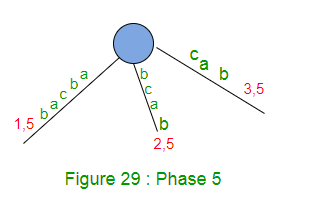At the end of phase 5, remainingSuffixCount is 2 (Two suffixes, ‘ab’ and ‘b’, the last two, are not added explicitly in tree, but they are in tree implicitly).

*********************Phase 6*********************************
In phase 6, we read 6th character (x) from string S

• Set END to 6 (This will do extensions 1, 2 and 3)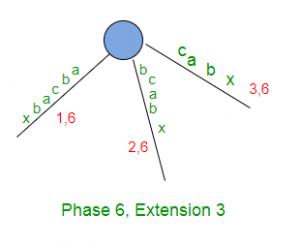• Increment remainingSuffixCount by 1 (remainingSuffixCount will be 3 here, i.e. there are 3 extension left to be performed, which are extensions 4, 5 and 6 for suffixes “abx”, “bx” and “x” respectively)
• Run a loop remainingSuffixCount times (i.e. three times) as below:
• While extension 4, the activePoint is (root, a, 2) which points to ‘b’ on edge starting with ‘a’.
• In extension 4, current character ‘x’ from string S doesn’t match with the next character on the edge after activePoint, so this is the case of extension rule 2. So a leaf edge is created here with edge label x. Also here traversal ends in middle of an edge, so a new internal node also gets created at the end of activePoint.
• Decrement the remainingSuffixCount by 1 (from 3 to 2) as suffix “abx” added in tree.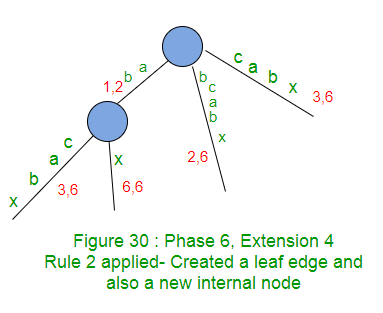Now activePoint will change after applying rule 2. Three other cases, (APCFER3, APCFWD and APCFALZ) where activePoint changes, are already discussed in Part 3.

activePoint change for extension rule 2 (APCFER2):
Case 1 (APCFER2C1): If activeNode is root and activeLength is greater than ZERO, then decrement the activeLength by 1 and activeEdge will be set “S[i – remainingSuffixCount + 1]” where i is current phase number. Can you see why this change in activePoint? Look at current extension we just discussed above for phase 6 (i=6) again where we added suffix “abx”. There activeLength is 2 and activeEdge is ‘a’. Now in next extension, we need to add suffix “bx” in the tree, i.e. path label in next extension should start with ‘b’. So ‘b’ (the 5th character in string S) should be active edge for next extension and index of b will be “i – remainingSuffixCount + 1” (6 – 2 + 1 = 5). activeLength is decremented by 1 because activePoint gets closer to root by length 1 after every extension.
What will happen If activeNode is root and activeLength is ZERO? This case is already taken care by APCFALZ.

Case 2 (APCFER2C2): If activeNode is not root, then follow the suffix link from current activeNode. The new node (which can be root node or another internal node) pointed by suffix link will be the activeNode for next extension. No change in activeLength and activeEdge. Can you see why this change in activePoint? This is because: If two nodes are connected by a suffix link, then labels on all paths going down from those two nodes, starting with same character, will be exactly same and so for two corresponding similar point on those paths, activeEdge and activeLength will be same and the two nodes will be the activeNode. Look at Figure 18 in Part 2. Let’s say in phase i and extension j, suffix ‘xAabcdedg’ was added in tree. At that point, let’s say activePoint was (Node-V, a, 7), i.e. point ‘g’. So for next extension j+1, we would add suffix ‘Aabcdefg’ and for that we need to traverse 2nd path shown in Figure 18. This can be done by following suffix link from current activeNode v. Suffix link takes us to the path to be traversed somewhere in between [Node s(v)] below which the path is exactly same as how it was below the previous activeNode v. As said earlier, “activePoint gets closer to root by length 1 after every extension”, this reduction in length will happen above the node s(v) but below s(v), no change at all. So when activeNode is not root in current extension, then for next extension, only activeNode changes (No change in activeEdge and activeLength).

• At this point in extension 4, current activePoint is (root, a, 2) and based on APCFER2C1, new activePoint for next extension 5 will be (root, b, 1)
• Next suffix to be added is ‘bx’ (with remainingSuffixCount 2).
• Current character ‘x’ from string S doesn’t match with the next character on the edge after activePoint, so this is the case of extension rule 2. So a leaf edge is created here with edge label x. Also here traversal ends in middle of an edge, so a new internal node also gets created at the end of activePoint.
Suffix link is also created from previous internal node (of extension 4) to the new internal node created in current extension 5.
• Decrement the remainingSuffixCount by 1 (from 2 to 1) as suffix “bx” added in tree.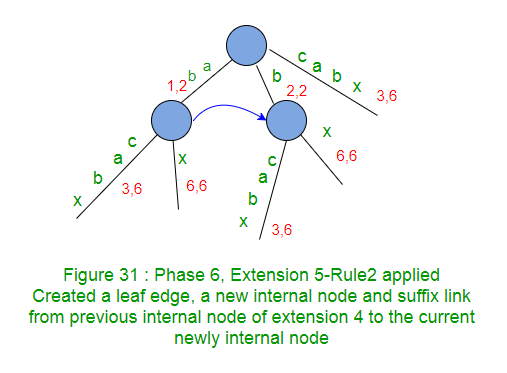• At this point in extension 5, current activePoint is (root, b, 1) and based on APCFER2C1 new activePoint for next extension 6 will be (root, x, 0)
• Next suffix to be added is ‘x’ (with remainingSuffixCount 1).
• In the next extension 6, character x will not match to any existing edge from root, so a new edge with label x will be created from root node. Also suffix link from previous extension’s internal node goes to root (as no new internal node created in current extension 6).
• Decrement the remainingSuffixCount by 1 (from 1 to 0) as suffix “x” added in tree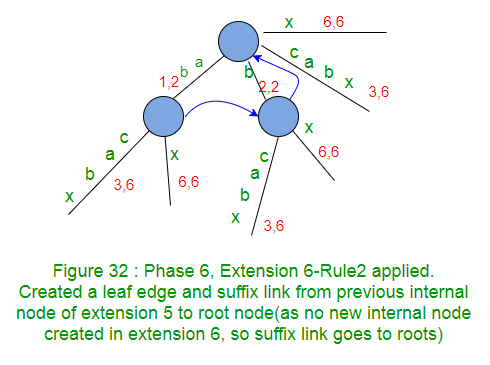This completes the phase 6.
Note that phase 6 has completed all its 6 extensions (Why? Because the current character c was not seen in string so far, so rule 3, which stops further extensions never got chance to get applied in phase 6) and so the tree generated after phase 6 is a true suffix tree (i.e. not an implicit tree) for the characters ‘abcabx’ read so far and it has all suffixes explicitly in the tree.

While building the tree above, following facts were noticed:

• A newly created internal node in extension i, points to another internal node or root (if activeNode is root in extension i+1) by the end of extension i+1 via suffix link (Every internal node MUST have a suffix link pointing to another internal node or root)
• Suffix link provides short cut while searching path label end of next suffix
• With proper tracking of activePoints between extensions/phases, unnecessary walkdown from root can be avoided.

We will go through rest of the phases (7 to 11) in Part 5 and build the tree completely and after that, we will see the code for the algorithm in Part 6.